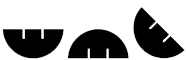#WUHAN MACHINE EQUIPMENT CO LTD

 WME >> Help >> Measure Exchange

# Measure Exchange

 Power: 1hp=0.746KW hp means horse power, and KW means kilowatt.       Length: 1"=25.4mm 1cm=0.394" mm means millimetre, and cm means centimetre. 1" means 1 inch.   1nm=0.001um 1um=0.001mm nm means nanometre, and um means micron.   1si=10um   1mm=0.001M 1cm=0.01M   1ft=30.5cm 1M=3.28ft ft means feet, and M means meter.   1yd=3ft=0.914M 1M=1.09yd yd means yard.   1mile=1.61KM 1KM=0.621mile KM means kilometer.   1hectometer=100M     Mass & Weight: 1carat=0.2g 1oz ≈ 31.1g 1g=0.03527oz g means gram, and oz means ounce.   1 lb=0.454KGS 1KGS=2.20 lb lb means pound, and KGS means kilograms   1MT=1000KGS MT means metric ton.   1KGS=9.8N       Area: 1km² = 1 000 000M² 1hm² = 1hectare = 1ha = 100are = 10000M² = 15mu (mou) 1acre ≈ 4047M² 1mu (mou) = 667M² 1yd² = 9ft² = 0.836M² 1ft² ≈ 0.093M² hm² means square hectometer.       Volume & Dimension: 1L=0.001M³ 1M³=1000L       Temperature: C=((F-32)*5)/9 F=(9*C)/5+32       Force: 1 KGS ≈ 10N 1 ton ≈ 10KN
 WUHAN MACHINE EQUIPMENT CO LTD © 2016